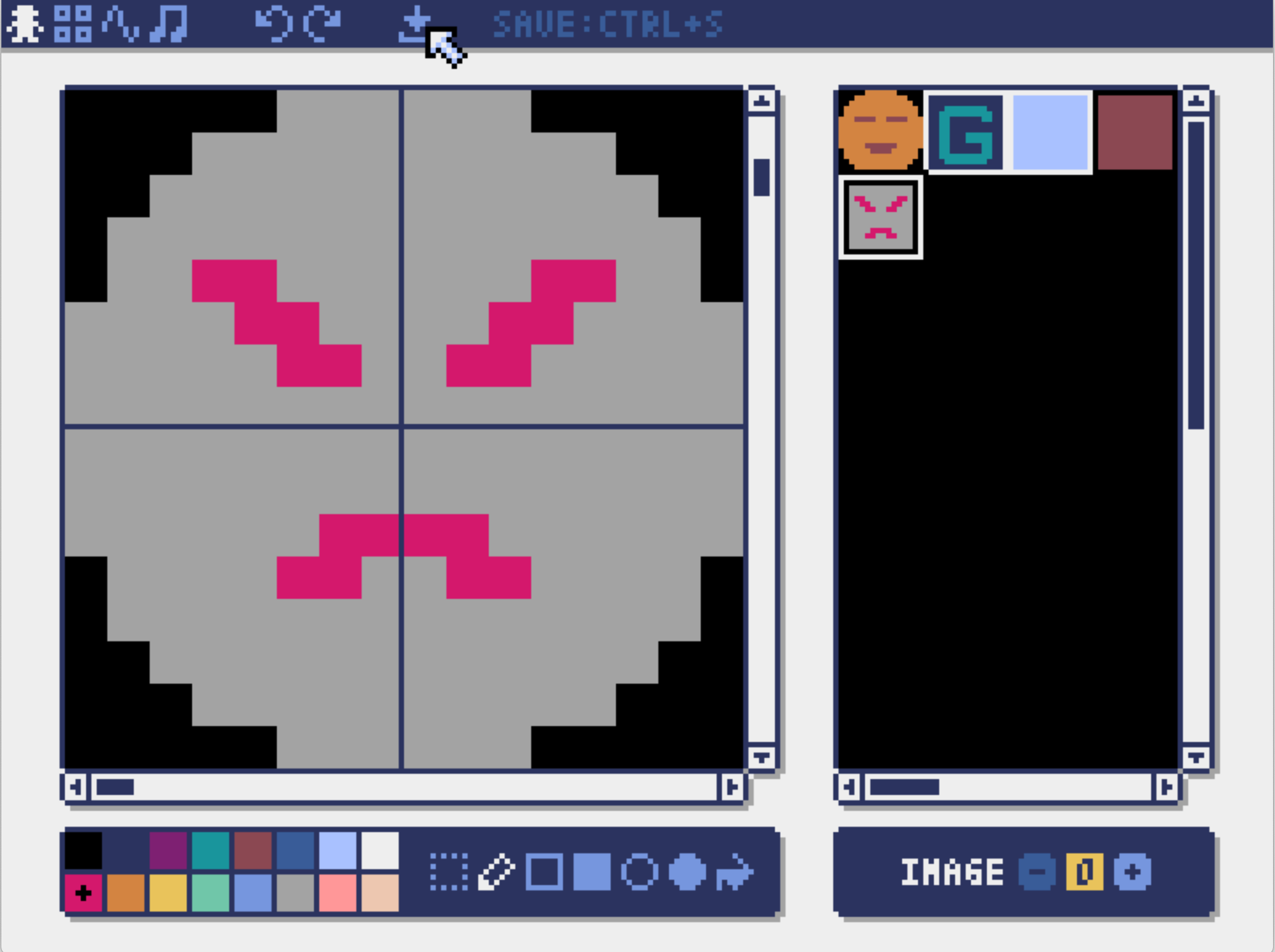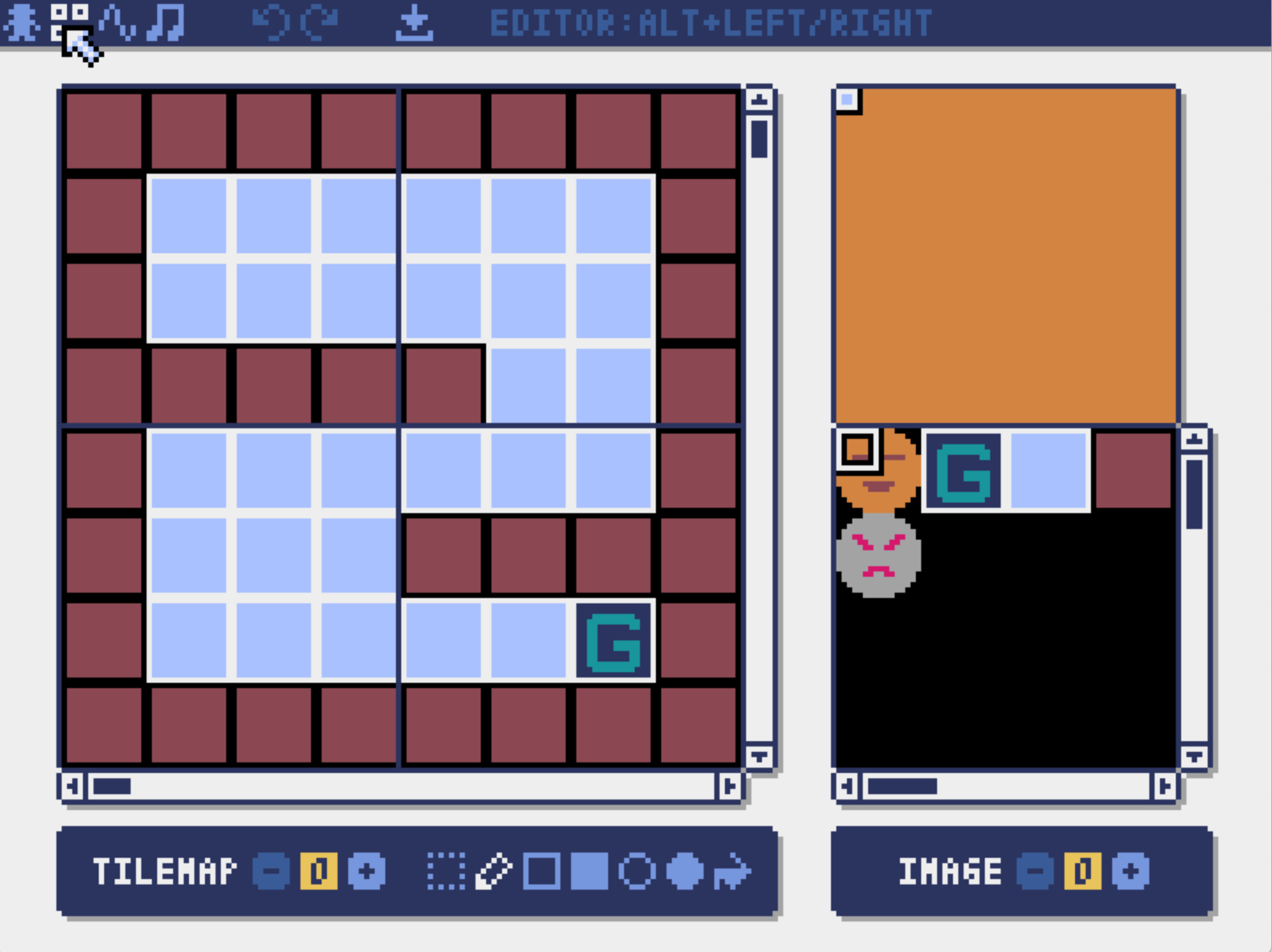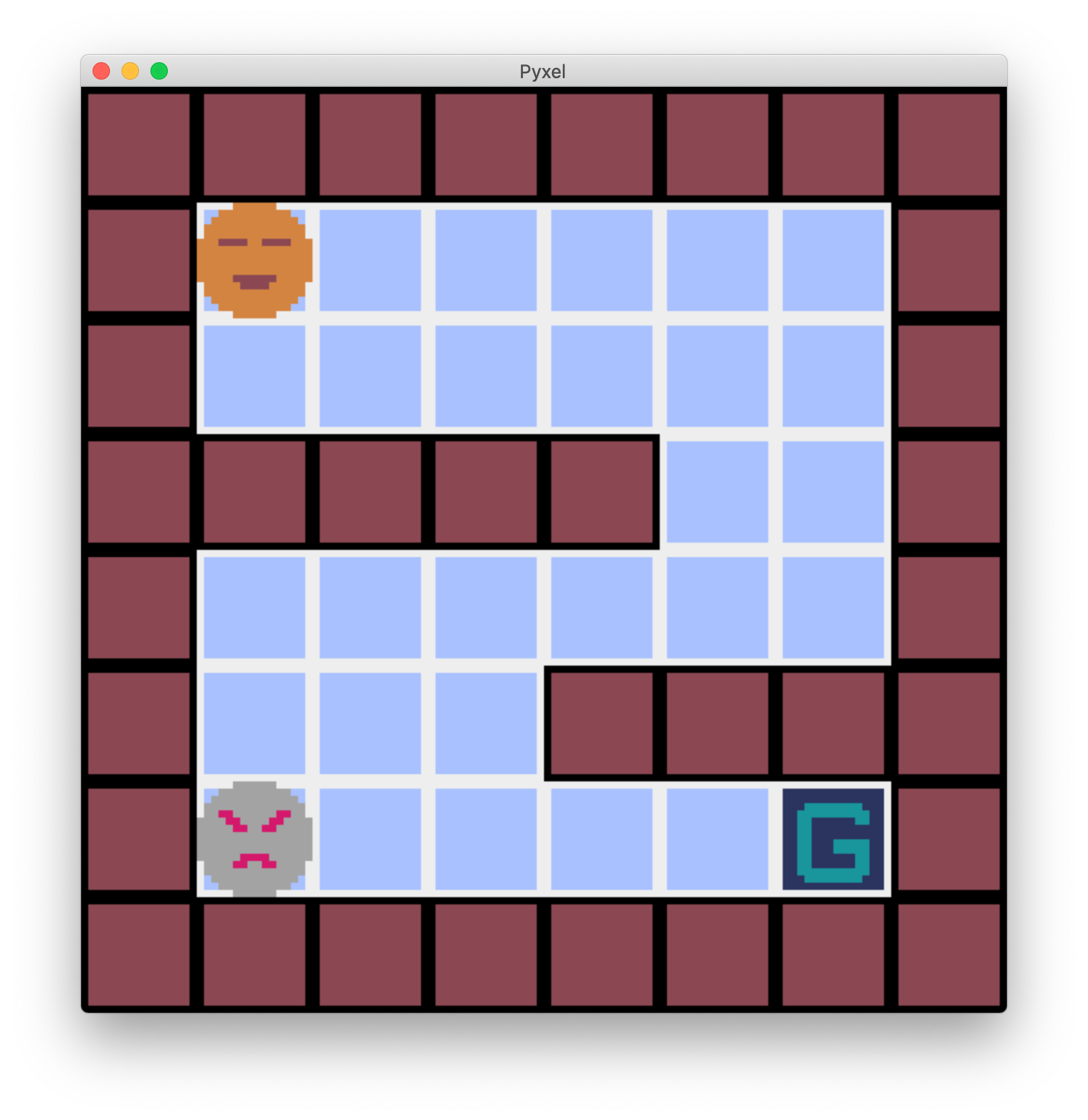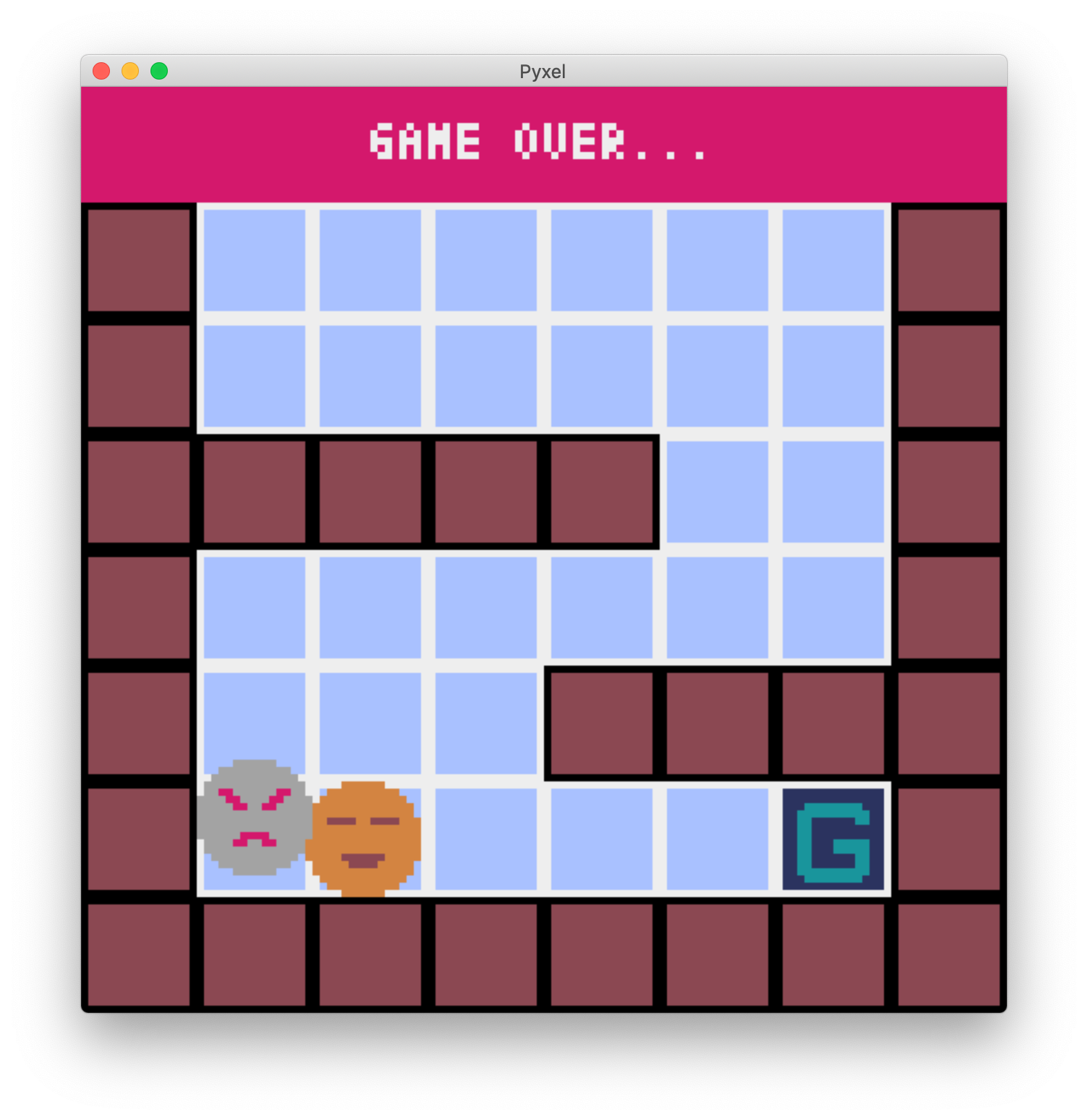# Introduction

Let's make an enemy character appear in a game that follows a maze and aims for a goal. I'd like to make it a little more game-like by making the game over when it hits.

# Preparation

Added an enemy character with a scary face.Increase the walls to make it look like a maze.The base code. The enemy hasn't appeared yet.

``````import pyxel

class Player:
def __init__(self, x, y):
self.x = x
self.y = y

self.dx = 0
self.dy = 0
self.move_count = 0

def move(self):
self.x += self.dx
self.y += self.dy

class App:
def __init__(self):
pyxel.init(128, 128)

self.player = Player(16, 16)

def run(self):
pyxel.run(self.update, self.draw)

def update_move(self):
if self.player.move_count == 0:
if pyxel.btnp(pyxel.KEY_LEFT):
self.player.dx = -1
elif pyxel.btnp(pyxel.KEY_RIGHT):
self.player.dx = 1
elif pyxel.btnp(pyxel.KEY_UP):
self.player.dy = -1
elif pyxel.btnp(pyxel.KEY_DOWN):
self.player.dy = 1

if self.player.dx != 0 or self.player.dy != 0:
self.player.move_count = 16

if pyxel.tilemap(0).get(
self.player.x / 8 + self.player.dx * 2,
self.player.y / 8 + self.player.dy * 2) == 6:
self.player.dx = 0
self.player.dy = 0
self.player.move_count = 0
else:
self.player.move()
self.player.move_count -= 1

if self.player.move_count == 0:
self.player.dx = 0
self.player.dy = 0

def update(self):
self.update_move()

def draw(self):
pyxel.cls(0)

pyxel.bltm(0, 0, 0, 0, 0, 16, 16, 0)

pyxel.blt(self.player.x, self.player.y, 0, 0, 0, 16, 16, 0)

if pyxel.tilemap(0).get(self.player.x / 8,self.player.y / 8) == 2:
pyxel.rect(0, 0, 128, 16, 8)
pyxel.text(40, 5, "GAME CLEAR!!", 7)

App().run()
``````

# Show enemies

``````class App:
def __init__(self):
pyxel.init(128, 128)

self.player = Player(16, 16)

#Generate an instance of an enemy character
self.enemy = Player(16, 96)
#Omission
def draw(self):
pyxel.cls(0)

pyxel.bltm(0, 0, 0, 0, 0, 16, 16, 0)

pyxel.blt(self.player.x, self.player.y, 0, 0, 0, 16, 16, 0)

#Drawing of enemy characters
pyxel.blt(self.enemy.x, self.enemy.y, 0, 0, 16, 16, 16, 0)
``````

Create an instance of the `Player` class to manage the coordinates of the enemy character. The y coordinate of the initial position is 128 at the bottom of the screen, so set it to 96, which is 2 squares higher than that. And add the function to draw the enemy in the drawing function. Of the arguments `(x, y, img, u, v, w, h, colkey)`, `x, y` specifies the variable of` enemy`, and `u, v` specifies` 16,16`. To do.# Move the enemy

The enemy will move randomly. The movement of the character is managed by the `update_move` function, so we will fix this. If you look closely, it seems that you can use it as it is except for the part of the first block that "determines the direction of movement by the pressed key". Therefore, cut out the part that "determines the direction of movement" as a separate function, and call different functions depending on whether your player moves or the enemy character moves. The function that "determines the direction of movement" is as follows. (Since the `random` module is used, write` import random` at the beginning.)

``````    #Your player decides the direction of movement by the key
def move_manual(self, player):
if pyxel.btnp(pyxel.KEY_LEFT):
player.dx = -1
elif pyxel.btnp(pyxel.KEY_RIGHT):
player.dx = 1
elif pyxel.btnp(pyxel.KEY_UP):
player.dy = -1
elif pyxel.btnp(pyxel.KEY_DOWN):
player.dy = 1

#Enemy characters randomly decide the direction of movement
def move_auto(self, player):
rand = random.randint(1, 4)
if rand == 1:
player.dx = -1
elif rand == 2:
player.dx = 1
elif rand == 3:
player.dy = -1
elif rand == 4:
player.dy = 1
``````

Then modify the `update_move` function. Until now, there was only one object of the `Player` class, so I was directly manipulating` self.player`. This time, I have two objects, myself and the enemy, so I will be able to select which object to move. So, I made an object of the `Player` class to move and a Boolean value indicating whether to move manually or automatically.

``````    def update_move(self, player, is_manual):
#Branch the function to call depending on whether it is manual movement or automatic movement
if player.move_count == 0:
if is_manual:
self.move_manual(player)
else:
self.move_auto(player)

#No change from here to below(However, self.Changing player to player)
if player.dx != 0 or player.dy != 0:
player.move_count = 16

if pyxel.tilemap(0).get(
player.x / 8 + player.dx * 2,
player.y / 8 + player.dy * 2) == 6:
player.dx = 0
player.dy = 0
player.move_count = 0
else:
player.move()
player.move_count -= 1

if player.move_count == 0:
player.dx = 0
player.dy = 0
``````

Finally, modify the inside of the `update` function.

``````    def update(self):
self.update_move(self.player, True)
self.update_move(self.enemy, False)
``````

The enemy character is now moving.

# Game over if you hit an enemy

If you hit an enemy, try to make the game over. Whether or not you have hit is determined by the relationship between the position coordinates of yourself and the enemy. It's the so-called "collision detection".

``````    #Determine if you hit an enemy
def check_hit(self, player, enemy):
dx = player.x - enemy.x
dy = player.y - enemy.y
if -16 < dx < 16 and -16 < dy < 16:
return True
else:
return False
``````

If both the x and y coordinates are between -16 and 16, it is judged that they have collided. (For the first time, I learned that you can write by connecting comparison operators like `-16 <dx <16`. It's convenient.) By the way, you can omit the argument and use `self.player` and` self.enemy`, but if there are multiple enemies, it will not be flexible, so I try to specify the object to be judged as the argument. ..

``````    def draw(self):
pyxel.cls(0)

pyxel.bltm(0, 0, 0, 0, 0, 16, 16, 0)

pyxel.blt(self.player.x, self.player.y, 0, 0, 0, 16, 16, 0)

pyxel.blt(self.enemy.x, self.enemy.y, 0, 0, 16, 16, 16, 0)

if pyxel.tilemap(0).get(self.player.x / 8,self.player.y / 8) == 2:
pyxel.rect(0, 0, 128, 16, 8)
pyxel.text(40, 5, "GAME CLEAR!!", 7)

#Game over if you hit an enemy
if self.check_hit(self.player, self.enemy):
pyxel.rect(0, 0, 128, 16, 8)
pyxel.text(40, 5, "GAME OVER...", 7)
``````

At the end of `draw`, I added a part to judge whether the game is over. If you hit an enemy with this, the game is over.

# Finish

As is the case when the game is cleared, the game will continue even if the game is over. When the game is cleared or the game is over, the game will end and it will not move.

``````    def __init__(self):
pyxel.init(128, 128)

self.player = Player(16, 16)

self.enemy = Player(16,96)

#Flag indicating the end of the game
self.game_end = False

#Omission
def update(self):
#Update only if the game is not over
if self.game_end == False:
self.update_move(self.player, True)
self.update_move(self.enemy, False)

def draw(self):
pyxel.cls(0)

pyxel.bltm(0, 0, 0, 0, 0, 16, 16, 0)

pyxel.blt(self.player.x, self.player.y, 0, 0, 0, 16, 16, 0)

#Drawing of enemy characters
pyxel.blt(self.enemy.x, self.enemy.y, 0, 0, 16, 16, 16, 0)

if pyxel.tilemap(0).get(self.player.x / 8,self.player.y / 8) == 2:
pyxel.rect(0, 0, 128, 16, 8)
pyxel.text(40, 5, "GAME CLEAR!!", 7)
self.game_end = True

#Game over if you hit an enemy
if self.check_hit(self.player, self.enemy):
pyxel.rect(0, 0, 128, 16, 8)
pyxel.text(40, 5, "GAME OVER...", 7)
self.game_end = True
``````

When the game was over, the update stopped and the game could not be continued.The difficulty level has become quite high. Below is the full code. It may be a little difficult to read because the function name is appropriate.

``````import pyxel
import random

class Player:
def __init__(self, x, y):
self.x = x
self.y = y

self.dx = 0
self.dy = 0
self.move_count = 0

def move(self):
self.x += self.dx
self.y += self.dy

class App:
def __init__(self):
pyxel.init(128, 128)

self.player = Player(16, 16)
self.enemy = Player(16, 96)

self.game_end = False

def run(self):
pyxel.run(self.update, self.draw)

def update_move(self, player, is_manual):
if player.move_count == 0:
if is_manual:
self.move_manual(player)
else:
self.move_auto(player)

if player.dx != 0 or player.dy != 0:
player.move_count = 16

if pyxel.tilemap(0).get(
player.x / 8 + player.dx * 2,
player.y / 8 + player.dy * 2) == 6:
player.dx = 0
player.dy = 0
player.move_count = 0
else:
player.move()
player.move_count -= 1

if player.move_count == 0:
player.dx = 0
player.dy = 0

def move_manual(self, player):
if pyxel.btnp(pyxel.KEY_LEFT):
player.dx = -1
elif pyxel.btnp(pyxel.KEY_RIGHT):
player.dx = 1
elif pyxel.btnp(pyxel.KEY_UP):
player.dy = -1
elif pyxel.btnp(pyxel.KEY_DOWN):
player.dy = 1

def move_auto(self, player):
rand = random.randint(1, 4)
if rand == 1:
player.dx = -1
elif rand == 2:
player.dx = 1
elif rand == 3:
player.dy = -1
elif rand == 4:
player.dy = 1

def check_hit(self, player, enemy):
dx = player.x - enemy.x
dy = player.y - enemy.y
if -16 < dx < 16 and -16 < dy < 16:
return True
else:
return False

def update(self):
if self.game_end == False:
self.update_move(self.player, True)
self.update_move(self.enemy, False)

def draw(self):
pyxel.cls(0)

pyxel.bltm(0, 0, 0, 0, 0, 16, 16, 0)

pyxel.blt(self.player.x, self.player.y, 0, 0, 0, 16, 16, 0)
pyxel.blt(self.enemy.x, self.enemy.y, 0, 0, 16, 16, 16, 0)

if pyxel.tilemap(0).get(self.player.x / 8,self.player.y / 8) == 2:
pyxel.rect(0, 0, 128, 16, 8)
pyxel.text(40, 5, "GAME CLEAR!!", 7)
self.game_end = True

if self.check_hit(self.player, self.enemy):
pyxel.rect(0, 0, 128, 16, 8)
pyxel.text(40, 5, "GAME OVER...", 7)
self.game_end = True

App().run()
``````